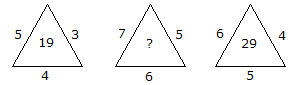# Verbal Reasoning - Character Puzzles - Discussion

### Discussion :: Character Puzzles - Character Puzzles 1 (Q.No.4)

4.

Which one will replace the question mark ?[A]. 25 [B]. 37 [C]. 41 [D]. 47

Explanation:

(5 x 3) + 4 = 19

and (6 x 4) + 5 = 29

Therefore, (7 x 5) + 6 = 41

 Dhaval Patel said: (Aug 26, 2010) (5 * 4) = 20, 20-1 = 19, (6 * 5) = 30, 30-1 = 29, Therefore, ( 7 * 6) = 42, 42-1= 41.

 Disha Sogani said: (Jan 28, 2011) It could be also solved as 4^2+3=19 5^2+4=29 Therefore,6^2+5=41

 Nandan said: (Apr 30, 2014) It could be also solved as, (5*4*3/3)-1, (6*5*4/4)-1, (7*6*5/5)-1.

 Momu said: (Aug 4, 2015) 4 square is 16+3=19. 5 square is 25+4=29. 6 square is 36+5=41.

 Sakshi said: (Oct 29, 2015) To simple! 5*3+4 = 19. 7*5+6 = 41. 6*4+5 = 29.

 Anil said: (Aug 30, 2017) (5 * 4) = 20, 20-1 = 19, (6 * 5) = 30, 30-1 = 29, Therefore, ( 7 * 6) = 42, 42-1= 41.

 Govardhan said: (Mar 29, 2020) 4*4=16, 16+3=19. 6*6=36, 36+5=41. 5*5=25, 25+4=29.